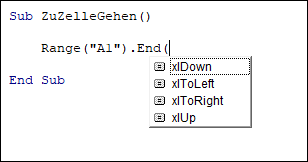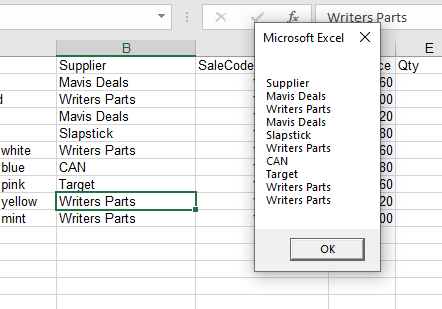# VBA Range.End (xlDown, xlUp, xlToRight, xlToLeft)

This tutorial will show you how to use the Range.End property in VBA.

Most things that you do manually in an Excel workbook or worksheet can be automated in VBA code.

If you have a range of non-blank cells in Excel, and you press Ctrl+Down Arrow, your cursor will move to the last non-blank cell in the column you are in.  Similarly, if you press Ctrl+Up Arrow, your cursor will move to the first non-blank cell.   The same applies for a row using the Ctrl+Right Arrow or Ctrl+Left Arrow to go to the beginning or end of that row.  All of these key combinations can be used within your VBA code using the End Function.

## Range End Property Syntax

The Range.End Property allows you to move to a specific cell within the Current Region that you are working with.

expression.End (Direction)

the expression is the cell address (Range) of the cell where you wish to start from eg: Range(“A1”)

END is the property of the Range object being controlled.

Direction is the Excel constant that you are able to use.  There are 4 choices available – xlDown, xlToLeft, xlToRight and xlUp.## Moving to the Last Cell

The procedure below will move you to the last cell in the Current Region of cells that you are in.

``````Sub GoToLast()
'move to the last cell occupied in the current region of cells
Range("A1").End(xlDown).Select
End Sub``````

## Counting Rows

The following procedure allows you to use the xlDown constant with the Range End property to count how many rows are in your current region.

``````Sub GoToLastRowofRange()
Dim rw As Integer
Range("A1").Select
'get the last row in the current region
rw = Range("A1").End(xlDown).Row
'show how many rows are used
MsgBox "The last row used in this range is " & rw
End Sub``````

While the one below will count the columns in the range using the xlToRight constant.

``````Sub GoToLastCellofRange()
Dim col As Integer
Range("A1").Select
'get the last column in the current region
col = Range("A1").End(xlToRight).Column
'show how many columns are used
MsgBox "The last column used in this range is " & col
End Sub``````

## Creating a Range Array

The procedure below allows us to start at the first cell in a range of cells, and then use the End(xlDown) property to find the last cell in the range of cells.  We can then ReDim our array with the total rows in the Range, thereby allowing us to loop through the range of cells.

``````Sub PopulateArray()
'declare the array
Dim strSuppliers() As String
'declare the integer to count the rows
Dim n As Integer
'count the rows
n = Range("B1", Range("B1").End(xlDown)).Rows.Count
'initialise and populate the array
ReDim strCustomers(n)
'declare the integer for looping
Dim i As Integer
'populate the array
For i = 0 To n
strCustomers(i) = Range("B1").Offset(i, 0).Value
Next i
'show message box with values of array
MsgBox Join(strCustomers, vbCrLf)
End Sub``````

When we run this procedure, it will return the following message box.Stop searching for VBA code online. Learn more about AutoMacro - A VBA Code Builder that allows beginners to code procedures from scratch with minimal coding knowledge and with many time-saving features for all users!